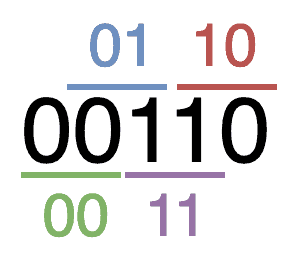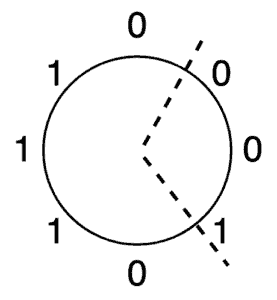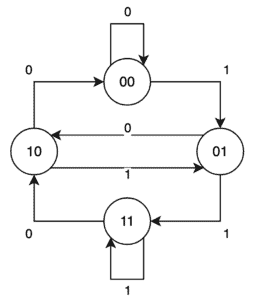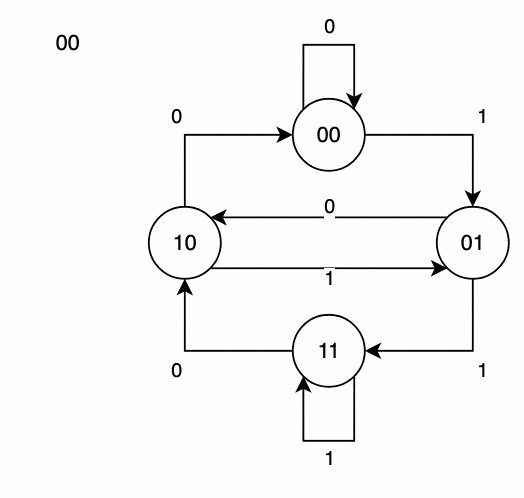## 1. Introduction

In this tutorial, we’re going to explore the de Bruijn sequence. We’ll see what it is, what it can be used for, and how to generate it ourselves.

## 2. What Is a de Bruijn Sequence?

A de Bruijn sequence is a mathematical construct. Given an alphabet with k elements, a de Bruijn sequence of order n is a sequence in which every possible string of length n from this alphabet appears exactly once.

This sounds more complicated than it really is, so we’ll break it down.

An “alphabet with k elements” means that we have this many unique symbols to deal with. For example, the English alphabet has 26 elements, and binary numbers can be considered an alphabet with 2 elements.

“Every possible string of length n” then means that we have every possible combination of n symbols from our alphabet. For example, if we take binary numbers and generate every string of length 2 then the results are 00, 01, 10, 11.

As such, a de Bruijn sequence is a single continuous sequence of symbols from our alphabet that contains each of these strings exactly once and nothing else.

For example, a de Bruijn sequence of order 2 for binary numbers would be:Considering the initial and final “0” symbols as being the same, we can see that this sequence is actually cyclical. That is, it can repeat forever, and we can start from anywhere in it, and it’ll still be the same. For example, this can be considered as “….00110011001100110011001100…..” as an infinite cyclical de Bruijn sequence.

## 3. Use Cases for de Bruijn Sequences

De Bruijn sequences have several uses in many fields, including but not limited to: Bioinformatics, Robotics, Data encryption, Information security, and many others.

### 3.1. Robotics

Robotics can make use of these sequences to identify the direction that a robot is facing. By generating a sequence and printing it in a circular pattern around a camera, it becomes possible to identify exactly which subsequence is visible and thus know which direction the camera is facing:This uses a de Bruijn sequence of order 3 for an alphabet of 2 symbols. The fact that it generates every possible sequence of 3 symbols in an overlapping structure means that we can print these symbols around our camera, as shown above. We can then read the 3 symbols that the camera can see at any point and know immediately which direction we’re facing.

If we do this with a suitable length sequence, then we can get a very high resolution with a relatively low size of the alphabet. For example, by reaching an order 5 sequence, we can uniquely identify 32 different directions with only 2 different values.

Keeping the size of our alphabet down can be very important in some situations. For example, if we’re using this for robotic guidance, our alphabet could be colours instead of symbols – “0” could be a black square and “1” a white square, which would then be easier for the camera to detect.

If we can increase the size of the alphabet, though, then the resolution goes up significantly. If we have 3 values in the alphabet, for example, Red, Green, and Blue, then a sequence of order 3 will allow us to identify 27 different directions uniquely, and by the time we get back to order 5, we have 243 unique directions.

### 3.2. Brute Forcing Locks

Imagine we have a lock with 10 unique buttons and a passcode that we know is 4 digits long, and it will unlock the instant we enter the correct 4 digits.

If we wanted to brute force this lock, we could start entering codes starting from “0000” and working our way up to “9999”. This will work but is inefficient – it would take up to 10,000 combinations which means up to 40,000 key presses.

Alternatively, we can generate a de Bruijn sequence of order 4 for an alphabet of size 10. This is a string that has every unique combination of symbols in it. As such, we can just start entering this sequence, and at some point, it’s guaranteed to contain the correct code.

The very fact that every code overlaps the previous one means that this sequence of key presses is guaranteed to be significantly shorter than just working our way from the start.

## 4. Generating de Bruijn Sequences

Now that we know what a de Bruijn sequence is and how it can be used, we need to know how to generate them. There is a relatively straightforward way to do this using some other techniques we’ve seen from graph theory.

We start by generating a set of nodes. If we’re working with a de Bruijn sequence of order k then each node contains a unique string of length k-1 from our alphabet. For example, given our alphabet of binary digits and a de Bruijn sequence of order 3, we would generate every unique string of length 2: 00, 01, 10, 11.

Having done this, we can then build a directed graph. Every node has an edge leaving it that joins it to the node that would be next in the de Bruijn sequence. For example, node “01” would join to “10” using an edge of “0”, and to “11” using an edge of “1”.

Every node has one edge for every symbol in our alphabet, though these will potentially lead back to the same node. This is fine and expected. For example, our graph here will look as follows:By definition, every one of our nodes will have one edge leaving it for every symbol in our alphabet, and an equally will have one edge entering it for every symbol in our alphabet. This means that we will always have an even number of edges for every node. This in turn means that we always have an Euler Path in our graph.

We can then make use of this to generate our de Bruijn sequence. We pick a node as our start, then follow the Euler Path throughout the entire graph, adding the appropriate symbol from that edge. Once we’ve traced the entire path we will have our de Bruijn sequence.

Note that as long as we end up on the same node that we started from then the path is guaranteed to be cyclical, which is what we want. Such a path is always guaranteed in the graph that we have constructed here because every node has an equal number of entry and exit edges.

For example, if we start at node “00” and follow edges “0”, “1”, “0”, “1”, “1”, “1”, “0”, “0” then we will have generated our sequence:### 4.1. Hierholzer’s Algorithm

Hierholzer’s algorithm is a technique that can be used to discover an Euler path in a graph. That is a path that traverses every edge on the graph exactly once and ends up on the same node that it started. If we can do this, then we can programmatically achieve what we discussed above – traverse every edge in our graph and add it to our sequence until we have generated the entire de Bruijn sequence.

Hierholzer’s algorithm relies on the fact that our graph has an equal number of entry and exit vertices from every node, and recursively builds circuits up until we have the entire path.

Firstly we need to generate the graph itself. We start by generating all of the nodes. This is every possible combination of k-1 symbols from our alphabet:

``

Then we need to generate the edges of our graph. To do this we need to find, for every node and every symbol in our alphabet, the node that it will connect to:

``

Finally, we can actually generate our sequence. This is done by selecting a node on our graph that has unused edges, and then following a circuit of unused edges until we get back to the starting node. We then repeat this until every edge has been used:

``

This looks complicated, so let’s work our way through it.

We start out by picking an arbitrary node in our graph – the choice doesn’t really matter. We then expand an entire sequence from this node, following arbitrary exit edges until we get back to our starting node. As we’re doing this, we remove every traversed edge from the list of future options.

Next, we pick an arbitrary node that is already on our sequence, as long as it has at least one unused exit node. This is then expanded in exactly the same way as above, and the newly expanded sequence is inserted into our result.

We can then repeat this process until there are no unused exits left. At this point, we have built up the complete sequence of nodes in the correct order, from which we can build our de Bruijn sequence.

At every step, our choice of nodes and edges is almost completely arbitrary. The only restriction is that we must pick nodes that have unused edges. This is because the initial graph has exactly the same number of entry and exit edges on every single node.

### 4.2. Working Example

Now we know how this works, let’s see it in action. We’ll use our graph from above to build the same sequence.

For our first pass, we arbitrarily select node “00”. From here, we follow edges “1”, “1”, “0”, “0” to end up back where we started, having a current sequence of “00”, “01”, “11”, “10”, “00”.

For our second pass, we need to select a node from this sequence that has unused exit edges. We’ll pick the first one – “00”. Expanding this, the only choice of edges to select from is “0”, which makes this sub-sequence “00”, “00”. Inserting this into our overall sequence gives us “00”, “00”, “01”, “11”, “10”, “00”.

Our next selected node will be “01”, giving us a sub-sequence of “01”, “10”, “01”. Inserting this into our overall sequence now gives “00”, “00”, “01”, “10”, “01”, “11”, “10”, “00”.

Finally, we select node “11”, which gives the sub-sequence of “11”, “11”, and inserting this gives an overall sequence of “00”, “00”, “01”, “10”, “01”, “11”, “11”, “10”, “00”.

At this point, we have no unused edges left, which means that we have our full sequence.

## 5. Summary

Here we’ve explored the concept of a de Bruijn sequence, including what they are and how to generate them ourselves. These sequences have a surprising number of uses, so why not try them out yourself?